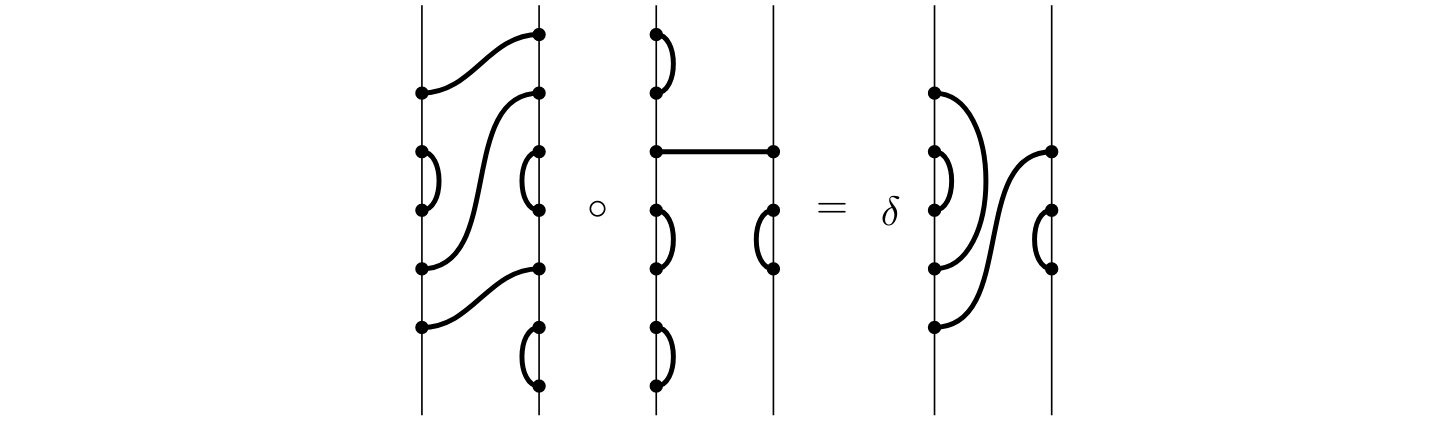# The Modular Temperley-Lieb Algebra

### Robert A. SpencerAbstract: We investigate the representation theory of the Temperley–Lieb algebra, $${\rm TL}_n(\delta)$$, defined over a field of positive characteristic. The principle question we seek to answer is the multiplicity of simple modules in cell modules for $${\rm TL}_n$$ over arbitrary rings. This provides us with the decomposition numbers for this algebra, as well as the dimensions of all simple modules. We obtain these results from diagrammatic principles, without appealing to realisations of $${\rm TL}_n$$ as endomorphism algebras of $$U_q(\mathfrak{sl}_2)$$ modules.

A draft of this work is available on the ArXiv.

#### Cite with BibTex

 @misc{spencer_2020_modular,
archiveprefix = "arXiv",
author = "Spencer, R. A.",
eprint = "2011.01328",
primaryclass = "math.RT",
title = "The Modular {T}emperley-{L}ieb Algebra",
year = "2020"
}